﻿ 一种基于贝叶斯和决策树的少数民族犯罪数据挖掘方法比较研究 Comparison of Various Classification Techniques for Ethnic Minority Crime Data Mining Based on Bayes and Decision Tree

Computer Science and Application
Vol. 09  No. 02 ( 2019 ), Article ID: 28850 , 12 pages
10.12677/CSA.2019.92039

Comparison of Various Classification Techniques for Ethnic Minority Crime Data Mining Based on Bayes and Decision Tree

Shaobing Wu1,2, Changmei Wang2*

1Institute of Information Security, Yunnan Police College, Kunming Yunnan

2Solar Energy Institute, Yunnan Normal University, Kunming Yunnan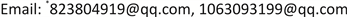Received: Jan. 28th, 2019; accepted: Feb. 6th, 2019; published: Feb. 13th, 2019ABSTRACT

Considering the slow speed and inconvenient operating of crime data mining method, we adopted three methods such as the use of Minitab, Bayes and decision trees to mine the data of a national crime area respectively. Then we compared the complexity, difficulty and accuracy of these three methods in mining crime law through concrete examples. In order to simplify calculation and facilitate practice, we designed program interface by C#. Experimental results showed that Bayesian approach performs better in predictive accuracy than Minitab and decision trees.

Keywords:Ethnic Minority Crime Data Mining, Bayes, Decision Tree, Minitab, Classification

1云南警官学院信息网络安全学院，云南 昆明

2云南师范大学太阳能研究所，云南 昆明1. 引言

2. 相关工作

3. 数据挖掘方法

3.1. 数据挖掘概述

3.2. 数据挖掘方法

3.2.1. 数据预处理

① 尽量放松清洗规则，保证数据的原样性；

② 在清洗过程中，只能作数据映射，不能修改用户数据，不对错误数据进行纠正；

③ 清洗的数据要保存。

1) 抽样

2) 降维

3) 去噪

3.2.2. 数据分析

3.2.3. 结果评价与展示

3.3. 数据挖掘算法

3.3.1. Minitab

3.3.2. 贝叶斯犯罪挖掘算法

$P\left(X|{C}_{i}\right)$ 被称为先验概率，是根据以往经验获得的在已知状态 ${C}_{i}$ 的情况下 ${X}_{i}$ 的分布。对于企业创新风险。先验概率就是在以往经历的失败或者成功的创新活动中，各风险指标的分布情况。在风险指标为离散值的情况下，先验概率可以通过统计历史数据的方法得到。

$P\left({C}_{i}|X\right)\text{}=\frac{P\left(X|{C}_{i}\right)P\left({C}_{i}\right)}{\sum _{j=1}^{n}P\left(X|{C}_{j}\right)P\left({C}_{j}\right)}$ (1)

3.3.3. 决策树

4. 基于Minitab、贝叶斯与决策树的犯罪数据挖掘应用研究

4.1. 基于Minitab对犯罪数据的挖掘

Minitab软件是由1972年成立于美国宾夕法尼亚大学的Minitab Inc.公司开发的。目前从新兴企业到全球500强公司大都选择Minitab作为改善流程、提高质量的首选软件，它是现代质量管理统计的领先者，是六西格玛管理中必需的统计分析软件，更是持续质量改进的良好工具软件，它的核心功能就是进行数据分析、图形分析以及趋势预测。本文使用Minitab 16.0版作为分析辅助工具，主要使用里面的回归分析模块。Table 1. Sample data of a national police crime analysisTable 2. Crime data coding table for a certain ethnic area

$Y={\beta }_{0}+\sum _{i=1}^{7}{\beta }_{i}{x}_{i}+\sum _{i=1}^{7}{\beta }_{ii}{x}_{i}^{2}+\sum _{i=1}^{2}\sum _{j=1}^{7}{\beta }_{ij}{x}_{i}{x}_{j}$ (2)Table 3. Estimated regression coefficients for y

$\begin{array}{l}S=0,\text{PRESS}=*\\ R-Sq=100.00%,R-Sq\left(预测\right)=*%R-Sq\left(调整\right)=100.00%\end{array}$

Table 4. Analysis of variance of y

 (3)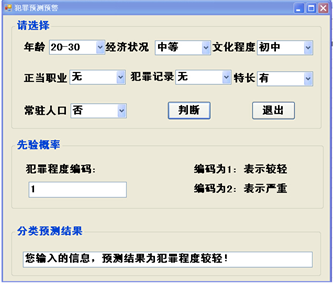Figure 1. Crime prediction based on Minitab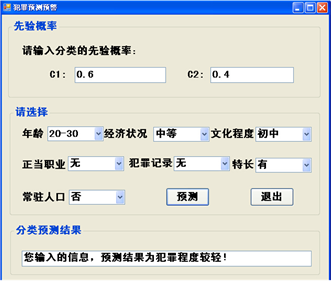Figure 2. Bayesian-based crime prediction

4.2. 基于贝叶斯方法的犯罪数据挖掘应用

P (年龄 = 20~30|犯罪程度 = 较轻) = 0.5；P (年龄 = 20~30|犯罪程度 = 较重) = 0.74；

P (经济状况 = 中等|犯罪程度 = 较轻) = 0.333；P (经济状况 = 中等|犯罪程度 = 较重) = 0.25；

P (文化程度 = 初中|犯罪程度 = 较轻) = 0.501；P (文化程度 = 初中|犯罪程度 = 较重) = 0.25；

P (正当职业 = 无|犯罪程度 = 较轻) = 0.333；P (正当职业 = 无|犯罪程度 = 较重) = 0.5；

P (犯罪记录 = 无|犯罪程度 = 较轻) = 0.667；P (犯罪记录 = 无|犯罪程度 = 较重) = 0.25；

P (特长 = 有|犯罪程度 = 较轻) = 0.167；P (特长 = 有|犯罪程度 = 较重) = 0.5；

P (常驻人口 = 是|犯罪程度 = 较轻) = 0.5；P (常驻人口 = 是|犯罪程度 = 较重) = 0.25；

$S=\sum _{j=1}^{n}P\left(X|{C}_{j}\right)P\left({C}_{j}\right)$ (4)

$P\left({C}_{1}|X\right)=\frac{P\left(X|{C}_{1}\right)P\left({C}_{1}\right)}{\sum _{j=1}^{n}P\left(X|{C}_{j}\right)P\left({C}_{j}\right)}=P\left(X|{C}_{1}\right)P\left({C}_{1}\right)/S$ (5)Table 5. Analysis of criminal behavior in a certain ethnic area

$P\left({C}_{2}|X\right)=0.\text{75}×0.\text{25}×0.\text{25}×0.\text{5}×0.\text{25}×0.\text{5}×0.\text{25}×0.\text{4}=0.000\text{292968}$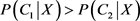Table 6. Criminal behavior analysis sample data conditional probability table

4.3. 基于决策树算法的犯罪数据挖掘应用

4.3.1. 选取挖掘目标和采集数据

4.3.2. 决策树算法的数据挖掘过程

1) 决策树模型的分类原理

a) 决策树的枝干和叶节点是如何生成的？

b) 节点的选取

i) 划分节点时，模型所需的信息量最小

ii) 能生成一个相对简洁的树

c) 基于信息熵的原理

2) 类别熵

$\text{H}\left(\text{C}\right)=-\sum _{i=1}^{k}{P}_{ci}{I}_{ci}=-\sum _{i=1}^{k}{P}_{ci}{\mathrm{log}}_{2}^{{P}_{ci}}$ (6)



$H\left(C\right)=H\left({C}_{1}\right)+H\left({C}_{2}\right)=\left(-0.6{\mathrm{log}}_{2}^{0.6}\right)+\left(-0.4{\mathrm{log}}_{2}^{0.4}\right)=0.306495+0.366516=0.670311$

3) 条件熵

$H\left(C|{X}_{1}\right)=-\sum _{i=1}^{k}{P}_{X1i}H\left(C|{X}_{i1}\right)$ (7)

$H\left(C|{X}_{12}\right)=\left(-0.667{\mathrm{log}}_{0}^{0.667}\right)+\left(-0.333{\mathrm{log}}_{2}^{0.333}\right)=0.270112+0.366171=0.636283$

$H\left(C|{X}_{13}\right)=0$

4) 信息增益

 (8)

$\text{Gain}\left(年龄\right)=H\left(C\right)-H\left(C|年龄\right)=0.670311-0.44458=0.225102$

$\text{Gain}\left(年龄\right)=\text{}H\left(C\right)-H\left(C|年龄\right)=0.670311-0.44458=0.225102$

$\text{Gain}\left(经济状况\right)=H\left(C\right)-H\left(C|经济状况\right)=0.\text{67}0\text{311}-0.\text{253696}=0.\text{416615}$

$\text{Gain}\left(文化程度\right)=H\left(C\right)-H\left(C|文化程度\right)=0.\text{67}0\text{311}-0.\text{4498688}=0.\text{22}0\text{4422}$

$\text{Gain}\left(正当职业\right)=H\left(C\right)-H\left(C|正当职业\right)=0.\text{67}0\text{311}-0.\text{588851}=0.0\text{8146}$

$\text{Gain}\left(犯罪记录\right)=H\left(C\right)-H\left(C|犯罪记录\right)=0.\text{67}0\text{311}-0.\text{64}0\text{8226}=0.0\text{294884}$

$\text{Gain}\left(特长\right)=H\left(C\right)-H\left(C|特长\right)=0.\text{67}0\text{311}-0.\text{588851}=0.0\text{8146}$

$\text{Gain}\left(常住人口\right)=H\left(C\right)-H\left(C|常住人口\right)=0.\text{67}0\text{311}-0.\text{6}0\text{98566}=0.0\text{6}0\text{4544}$

5) 决策树的生成Table 7. Information gain table with economic statusTable 8. Information gain table without economic status

4.3.3. 决策树挖掘规则应用

1) IF经济状况 = 中等，THEN犯罪程度 = 较轻；

2) IF经济状况 = 差and常驻人口 = 否，THEN犯罪程度 = 严重；

3) IF经济状况 = 差and常驻人口 = 是and正当职业 = 有，THEN犯罪程度 = 较轻；

4) IF经济状况 = 差and常驻人口 = 是and正当职业 = 无，THEN犯罪程度 = 严重；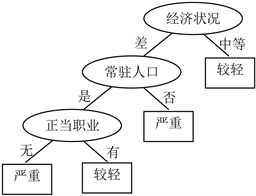Figure 3. Crime prediction decision tree

4.4. 三种犯罪数据挖掘方法的分析比较Table 9. Comparison of crime data mining in three methods

5. 结论

Comparison of Various Classification Techniques for Ethnic Minority Crime Data Mining Based on Bayes and Decision Tree[J]. 计算机科学与应用, 2019, 09(02): 339-350. https://doi.org/10.12677/CSA.2019.92039

1. 1. 乔少杰, 唐常杰, 彭京, 等. 基于个性特征仿真邮件分析系统挖掘犯罪网络核心[J]. 计算机学报, 2008, 31(10): 1795-1803.

2. 2. 金光, 钱家麒, 钱江波, 黄蔚民. 基于数据挖掘决策树的犯罪风险预测模型[J]. 计算机工程, 2003, 29(9): 183-185.

3. 3. 黄建设, 姚奇富. 数据挖掘技术在犯罪行为分析中的应用[J]. 浙江工商职业技术学院学报, 2005, 4(3): 45-47.

4. 4. 李万彪, 余志, 龚峻峰, 陈锐祥. 基于关系数据模型的犯罪网络挖掘研究[J]. 中山大学学报(自然科学版), 2014, 53(5): 1-7.

5. 5. 杨莉莉, 杨永川. 基于社会网络的犯罪组织关系挖掘[J]. 计算机工程, 2009, 35(15): 91-93.

6. 6. Oatley, G.C., Zeleznikow, J. and Ewart, B.W. (2004) Matching and Predicting Crimes. In: Macintosh, A., Ellis, R. and Allen, T., Eds., Applications and Innovations in Intelli-gent Systems XII in Proceedings of AI2004, The Twenty-Fourth SGAI International Conference on Knowledge Based Systems and Applications of Artificial Intelligence, Springer, London, 19-32.

7. 7. Adderley, R.W. (2007) The Use of Data Mining Techniques in Crime Trend Analysis and Offender Profiling. Ph.D. Thesis, University of Wolverhampton, Wolverhampton.

8. 8. 吴绍兵. 基于贝叶斯网络的刑事犯罪影响因素研究[J]. 计算机与数字工程, 2012, 227(11): 108-111.

9. 9. 张良均, 陈俊德, 刘名军, 陈荣. 数据挖掘实例分析[M], 北京: 机械工业出版社, 2015.

10. 10. Han, J.W., Kamber, M. and Pei, J. (2011) Data Mining: Concepts Andtech-niques. 3rd Edition, Elsevier Science, Burlington.

11. 11. Ricci, F., Rokach, L., Shapira, B., Kantor, P.B., 编. 推荐系统: 技术、评估及高效算法[M]. 李艳民, 胡聪, 吴宾, 王雪丽, 等, 译. 北京: 机械工业出版社, 2015: 33-35.

12. 12. Liu, W.Q. (2014) Modeling Data Quality Control System for Chinese Public Database and Its Empirical Analysis. Science China: Information Science, 44, 835-856.

13. 13. 米允龙, 米春桥, 刘文奇. 海量数据挖掘过程相关技术研究进展[J]. 计算机科学与探索, 2015, 9(6): 641-659.

14. 14. Mladenic, D. (1999) Text-Learning and Related Intelligent Agents: A Survey. IEEE Intelligent System, 14, 44-54. https://doi.org/10.1109/5254.784084

15. NOTES

*通讯作者。